## 7.4Turbulent boundary layers

At solid walls, the tangential ﬂow speed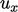increases rapidly across a thin boundary layer, as discussed in Sec. 6.4 . At high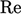, the velocity proﬁle has a universal character shown below.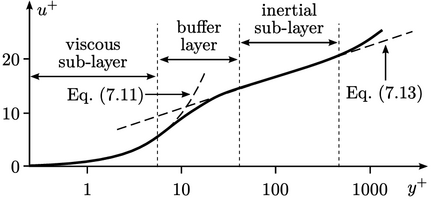The proﬁle compares measured data5 in terms of a dimensionless velocity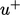and distance to the wall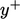, given by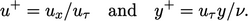(7.9)
Both parameters in Eq. (7.9 ) are based on a friction velocity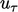which is related to the wall shear stress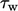by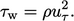(7.10)
At the wall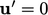. Close to the wall,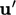is suppressed, creating a region where ﬂow is laminar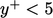, known as the viscous sub-layer . The proﬁle in this region is described by the relation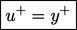(7.11)
Turbulence becomes signiﬁcant through the buﬀer layer which describes the region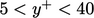. Van Driest provides a model for the increase in mixing length through this region, by6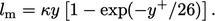(7.12)
Finally, in the inertial sub-layer for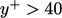, ﬂow is turbulent and the velocity proﬁle is described by the logarithmic law of the wall, often abbreviated to simply the log law, according to7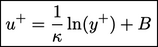(7.13)
The equation includes Kármán’s constant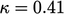and constant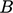. For a smooth wall,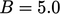8 – 5.5 is commonly used. Both Eq. (7.11 ) and Eq. (7.13) can be derived assuming a constant shear stress across the proﬁle, equating to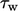at the wall. In the viscous sub-layer, the shear stress is laminar, so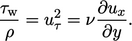(7.14)
This equation integrates with a zero constant of integration to give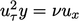, from which Eq. (7.11 ) is derived. In the inertial sub-layer, the shear stress is turbulent (laminar is negligible), so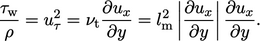(7.15)
Assuming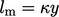gives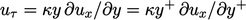, which integrates to yield Eq. (7.13). In the inertial sub-layer,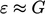as described in Eq. (7.5 ), which combines with Eq. (7.15 ) and Eq. (6.31 ) to give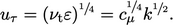(7.16)

5Joseph Kestin and Peter Richardson, Heat transfer across turbulent, incompressible boundary layers, 1963.
6Edward van Driest, On turbulent ﬂow near a wall, 1956.
7Alternatively written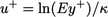, where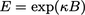.
8Hermann Schlichting and Klaus Gersten, Boundary-layer theory, 2017.

Notes on CFD: General Principles - 7.4 Turbulent boundary layers